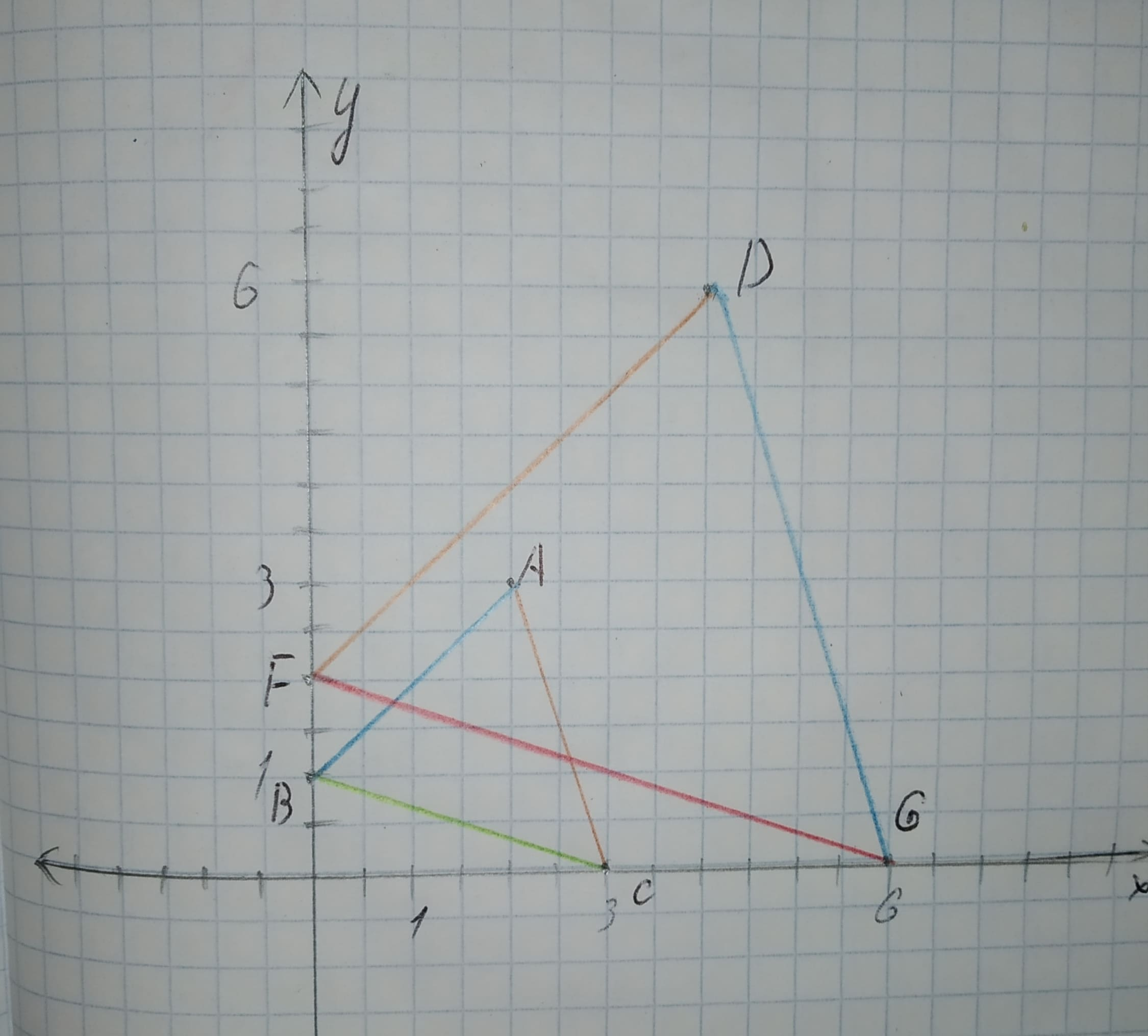# Draw a graph for the original figure and its dilated image. Check whether the dilation is a similarity transformation or not. Given: The given vertices are original rarr A(2,3),B(0,1),C(3,0) image rarr D(4,6),F(0,2),G(6,0)abondantQ 2020-10-27 Answered
Draw a graph for the original figure and its dilated image. Check whether the dilation is a similarity transformation or not.
Given:
The given vertices are
original $\to A\left(2,3\right),B\left(0,1\right),C\left(3,0\right)$
image $\to D\left(4,6\right),F\left(0,2\right),G\left(6,0\right)$
You can still ask an expert for help

• Questions are typically answered in as fast as 30 minutes

Solve your problem for the price of one coffee

• Math expert for every subject
• Pay only if we can solve itdavonliefI

Calculation:
The graph for the points is given below.Find the distance (using distance formula) of corresponding sides and find the ratio.
$AB=\sqrt{{\left(2-0\right)}^{2}+{\left(3-1\right)}^{2}}=\sqrt{8}=2\sqrt{2}$
$DF=\sqrt{{\left(4-0\right)}^{2}+{\left(6-2\right)}^{2}}=\sqrt{32}=4\sqrt{2}$
$\frac{AB}{DF}=\frac{2\sqrt{2}}{4\sqrt{2}}=\frac{1}{2}$
$AC=\sqrt{{\left(2-3\right)}^{2}+{\left(3-0\right)}^{2}}=\sqrt{10}$
$DG=\sqrt{{\left(4-6\right)}^{2}+{\left(6-0\right)}^{2}}=\sqrt{40}=2\sqrt{10}$
$\frac{AC}{DG}=\frac{\sqrt{10}}{2\sqrt{10}}=\frac{1}{2}$
$BC=\sqrt{{\left(0-3\right)}^{2}+{\left(1-0\right)}^{2}}=\sqrt{10}$
$FG=\sqrt{{\left(0-6\right)}^{2}+{\left(2-0\right)}^{2}}=\sqrt{40}=2\sqrt{10}$
$\frac{BC}{FG}=\frac{\sqrt{10}}{2\sqrt{10}}=\frac{1}{2}$
From the above result, the lenght of the sides are proportional.
By the use of SSS similarity,
$\mathrm{△}DFG\sim \mathrm{△}ABC$.
Hence the dilation is a similarity transformation.